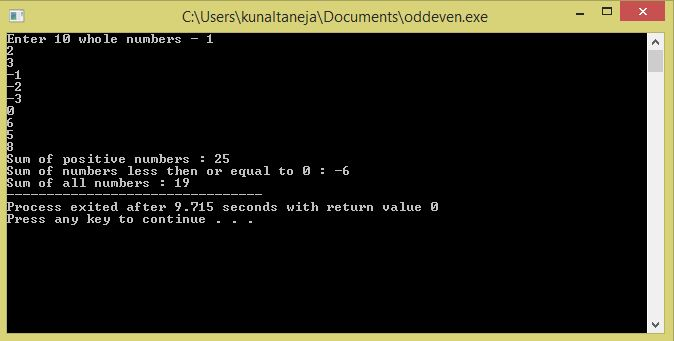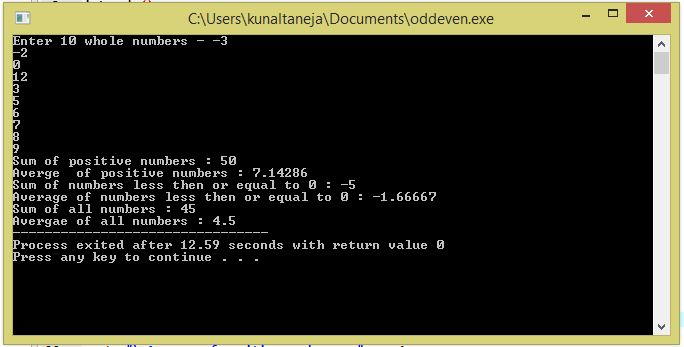NOTE: Please READ All Steps very carefully. DO #10! (10 is based off of 9).

Program Info:

#9. Write a program that reads in ten whole numbers and that outputs the sum of all the numbers greater than zero, the sum of all the numbers less than zero (which will be a negative number or zero), and the sum of all the numbers, whether positive, negative, or zero. The user enters the ten numbers just once each and the user can enter them in any order. Your program should not ask the user to enter the positive numbers and the negative numbers separately. 10. Modify your program from Programming Project 9 so that it outputs the sum of all positive numbers, the average of all positive numbers, the sum of all nonpositive numbers, the average of all nonpositive numbers, the sum of all positive and nonpositive numbers, and the average of all numbers entered.

#10. Modify your program from Programming ***Project 9*** so that it outputs the sum of all positive numbers, the average of all positive numbers, the sum of all nonpositive numbers, the average of all nonpositive numbers, the sum of all positive and nonpositive numbers, and the average of all numbers entered.

More Important Info:

You will need a counting loop that goes from 0 to 10.

Inside the loop, separate the positive and negative numbers using an if/else

maintain the total of the positive and negative numbers in the if/else

maintain the grand total in the body of the loop.

outside the loop, print the report of the total of the positive, negative and the grand total.

You should also count the number of the positive and negative numbers and print it at the end.

Document like your life depends on it!

9- #include<iostream>
using namespace std;

int main()
{
int arr;
int sum1=0,sum2=0,sum3=0;
cout<<“Enter 10 whole numbers – “;
for(int i=0;i<10;i++)
cin>>arr[i];
for(int i=0;i<10;i++)
{if(arr[i]>0)
sum1+=arr[i];
else
sum2+=arr[i];
}
sum3=sum1+sum2;
cout<<“Sum of positive numbers : “<<sum1;
cout<<“nSum of numbers less then or equal to 0 : “<<sum2;
cout<<“nSum of all numbers : “<<sum3;
}10-

#include<iostream>

using namespace std;

int main()

{

int arr;

int sum1=0,sum2=0,sum3=0,c1=0,c2=0;

float avg1,avg2,avg3;

cout<<“Enter 10 whole numbers – “;

for(int i=0;i<10;i++)

cin>>arr[i];

for(int i=0;i<10;i++)

{if(arr[i]>0){

++c1;

sum1+=arr[i];

}

else{

++c2;

sum2+=arr[i];

}

}

sum3=sum1+sum2;

avg1=(float)sum1/c1;

avg2=(float)sum2/c2;

avg3=(float)sum3/10;

cout<<“Sum of positive numbers : “<<sum1;

cout<<“nAverge of positive numbers : “<<avg1;

cout<<“nSum of numbers less then or equal to 0 : “<<sum2;

cout<<“nAverage of numbers less then or equal to 0 : “<<avg2;

cout<<“nSum of all numbers : “<<sum3;

cout<<“nAvergae of all numbers : “<<avg3;

}Pages (550 words)
Approximate price: -

Help Me Write My Essay - Reasons:Best Online Essay Writing Service

We strive to give our customers the best online essay writing experience. We Make sure essays are submitted on time and all the instructions are followed.Our Writers are Experienced and Professional

Our essay writing service is founded on professional writers who are on stand by to help you any time.Free Revision Fo all Essays

Sometimes you may require our writers to add on a point to make your essay as customised as possible, we will give you unlimited times to do this. And we will do it for free.Timely Essay(s)

We understand the frustrations that comes with late essays and our writers are extra careful to not violate this term. Our support team is always engauging our writers to help you have your essay ahead of time.Customised Essays &100% Confidential

Our Online writing Service has zero torelance for plagiarised papers. We have plagiarism checking tool that generate plagiarism reports just to make sure you are satisfied.Try it now!

## Calculate the price of your order

Total price:
\$0.00

How it works?Fill in the order form and provide all details of your assignment.Proceed with the payment

Choose the payment system that suits you most.HOW OUR ONLINE ESSAY WRITING SERVICE WORKS

Let us write that nagging essay.By clicking on the "PLACE ORDER" button, tell us your requires. Be precise for an accurate customised essay. You may also upload any reading materials where applicable.Pick A & Writer

Our ordering form will provide you with a list of writers and their feedbacks. At step 2, its time select a writer. Our online agents are on stand by to help you just in case.Editing (OUR PART)

At this stage, our editor will go through your essay and make sure your writer did meet all the instructions.